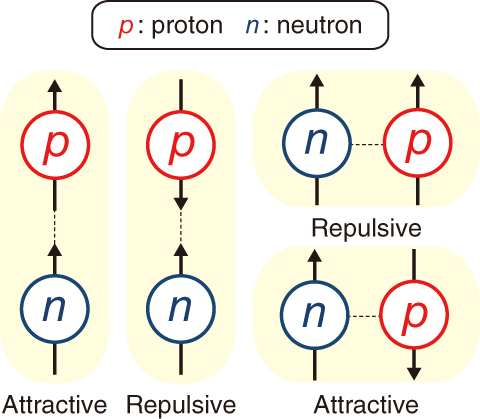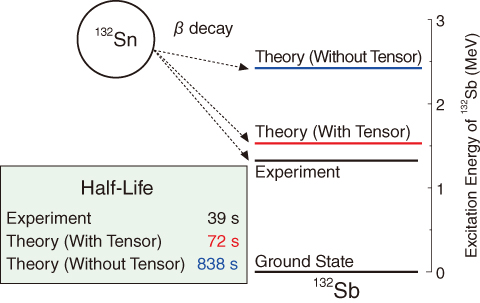# 4-1 Improving the Prediction Accuracy of the Decay Heat from Nuclear Force

## －Clarify Effect of Tensor Force on β Decay－Fig.4-3　Interaction due to tensor force between proton and neutron

The tensor force is either attractive or repulsive depending on the direction of the “spin” (spin axis is represented by arrows in the figure) and the relative positions of the proton and neutron. The attractive tensor force plays an important role in β decay.Fig.4-4　Half-life of 132Sn and excitation energy of daughter nucleus 132Sb with and without tensor force

The horizontal lines in the figure indicate the excitation energy of 132Sb. By considering the tensor force, the half-life of 132Sn and the excitation energy of 132Sb obtained by theory approach the experimental results.

Fission products (FPs) generated in nuclear power plants and fragments produced by nuclear disintegration reactions in accelerators contain unstable nuclei. Unstable nuclei typically change into more stable nuclei by β decay. β decay involves emission of an electron (positron), an antineutrino (neutrino), and a photon, and delivers energy to the environment. The energy released in β decay of a single nucleus is very small. However, spent nuclear fuel contains an enormous quantity of FPs, which produces a large amount of heat (decay heat). The decay heat is one of the problems that complicate the reprocessing of spent nuclear fuel and the disposal of radioactive waste. In addition, decay heat is the main reason that lots of heat is generated in the damaged nuclear reactors of the TEPCO’s Fukushima Daiichi NPS. However, some aspects of β decay remain unexplained by current theoretical models. In particular, theoretical models cannot reproduce energy by β decay or the half-life of nuclei with magic numbers. This fact reduces the accuracy predicting decay heat.

To solve this problem, we focus on the tensor force, which is not accounted for in the conventional models. The tensor force is one of the fundamental nuclear forces that govern the interaction between protons and neutrons, the constituents of the nucleus. Fig.4-3 shows the characteristic feature of the tensor force. The tensor force is either attractive or repulsive depending on the “spin” of the particles involved. Spin is analogous to the rotation of the particle and the relative position of proton and neutron. We developed a new theoretical model that includes the tensor force and numerically analyzed it to study how the tensor force affects β decay. We found that the attractive tensor force plays an important role in β decay. As shown in Fig.4-4, the half-life of the magic-number nucleus, tin-132 (132Sn), approaches the experimental result and the excitation energy of its daughter nucleus, antimony-132 (132Sb), is accurately reproduced.

This result contributes to improving the accuracy of predicting decay heat and should be useful in various fields of research, such as cosmic nucleosynthesis and fundamental research in nuclear physics.

| | | | |